# Polynomial Regression in Machine Learning

In this page, we will learn What is Polynomial Regression in Machine Learning?, Need for Polynomial Regression, Implementation of Polynomial Regression using Python, Steps for Polynomial Regression, Data Pre-Processing Step, Building the Linear regression model, Building the Polynomial regression model, Visualizing the result for Linear regression, Using the Linear Regression model to predict the final result.

## What is Polynomial Regression in Machine Learning?

• Polynomial Regression is a regression approach that uses an nth degree polynomial to represent the connection between a dependent(y) and independent variable(x). The equation for polynomial regression is as follows:

``````
y = b0+b1x1+ b2x12+ b2x13+...... bnx1n
```
```
• In machine learning, it's also known as the specific case of Multiple Linear Regression. Because we turn the Multiple Linear regression equation into Polynomial Regression by adding certain polynomial terms.
• It's a linear model that's been tweaked a little to improve accuracy.
• The training dataset for polynomial regression is non-linear in character.
• To fit the intricate and non-linear functions and datasets, it employs a linear regression model.

Hence, "In Polynomial regression, the original features are converted into Polynomial features of required degree (2,3,..,n) and then modeled using a linear model."

## Need for Polynomial Regression:

The importance of polynomial regression in machine learning can be shown in the following points:

• When we apply a linear model to a linear dataset, we get a nice result, as we saw in Simple Linear Regression, but when we apply the same model to a non-linear dataset without any modifications, we get a drastic outcome. As a result of the increased loss function, the error rate will be high, and accuracy will be reduced.
• In such instances, where data points are ordered non-linearly, the Polynomial Regression model is required. The following comparison diagram of the linear and non-linear datasets will help us comprehend it better.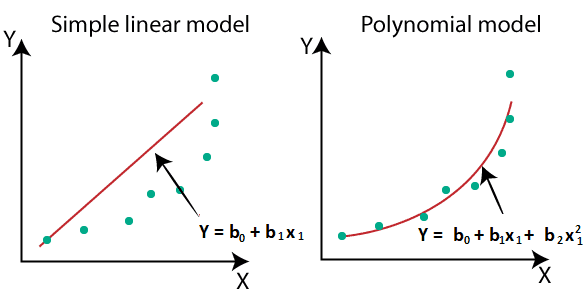• We used a dataset that was organized non-linearly in the image above. So, if we use a linear model to cover it, we can see that it barely covers any data points. The Polynomial model, on the other hand, uses a curve to encompass the majority of the data points.
• As a result, if the datasets are structured non-linearly, we should utilize the Polynomial Regression model rather than the Simple Linear Regression model.

Note: A Polynomial Regression algorithm is sometimes known as Polynomial Linear Regression since it is based on coefficients that are ordered in a linear form rather than variables.

Equation of the Polynomial Regression Model:       y = b0+b1x         .......(a)

Multiple Linear Regression equation:       y = b0+b1x+ b2x2+ b3x3+....+ bnxn       ....(b)

Polynomial Regression equation:         y = b0+b1x + b2x2+ b3x3+....+ bnxn      ....(c)

When we compare the three equations above, we can see that they are all polynomial equations, but the degree of variables differs. Polynomial equations with a single degree are also Simple and Multiple Linear equations, while the Polynomial regression equation is a Linear equation with the nth degree. Our linear equations will be changed to Polynomial Linear equations if we add a degree to them.

Note: You must be familiar with Simple Linear Regression in order to comprehend Polynomial Regression.

## Implementation of Polynomial Regression using Python:

We will use Python to implement Polynomial Regression. By contrasting the Polynomial Regression model with the Simple Linear Regression model, we will be able to comprehend it. So, initially, let's define the problem for which the model will be developed.
The problem is that there is a Human Resource firm that is going to hire a new employee. The candidate has stated that his prior pay was 160K per year, and HR must determine whether he is speaking the truth or making a bluff. So far, all they have is a dataset from his prior employer, which lists the wages of the top ten positions along with their levels. We discovered a non-linear association between the Position levels and salaries by examining the dataset available. Our goal is to create a Bluffing Detection Regression Model that will allow HR to pick a trustworthy candidate. The steps for making such a model are outlined below.

Position Level(X-variable) Salary(Y-variable)
Jonior Consultant 2 50,000
Senior Consultant 3 80,000
Manager 4 110,000
Country Manager 5 200,000
Region Manager 6 300,000
Partner 7 500,000
Senior Partner 8 330,000
C-Level 9 500,000
CEO 10 1000,000

## Steps for Polynomial Regression:

The main steps involved in Polynomial Regression are given below:

• Data Pre-processing
• Build a Linear Regression model and fit it to the dataset
• Build a Polynomial Regression model and fit it to the dataset
• Visualize the result for Linear Regression and Polynomial Regression model.
• Predicting the output.

Note: We'll design a Linear Regression model as well as a Polynomial Regression model to examine how the forecasts compare. In addition, there is a linear regression model for reference.

## Data Pre-Processing Step:

With a few exceptions, the data pre-processing procedure will be the same as in previous regression models. We will not employ feature scaling in the Polynomial Regression model, and we will not partition our dataset into training and test sets. There are two reasons for this:

• Our model will not be able to discover correlations between wages and levels if the dataset is divided into a test and training set because it contains very little information.
• We want very precise salary projections in our model, so it needs to have a lot of data.

The following is the code for the pre-processing step:

``````
#importing libraries
import numpy as nm
import matplotlib.pyplot as mtp
import pandas as pd

#importing datasets

#Extracting Independent and dependent Variable
x = data_set.iloc[:, 1:2].values
y = data_set.iloc[:, 2].values
```
```

## Explanation:

• We have imported the necessary Python libraries to import and act on the dataset in the given lines of code.
• Next, we've imported the 'Position Salaries.csv' dataset, which has three columns (Position, Levels, and Salary), but we'll simply look at the first two (Salary and Levels).
• The dependent (Y) and independent (X) variables were then retrieved from the dataset. We used the parameters [:,1:2] for the x-variable because we only want one index(levels) and :2 to make it a matrix.

Output:
By executing the above code, we can read our dataset as: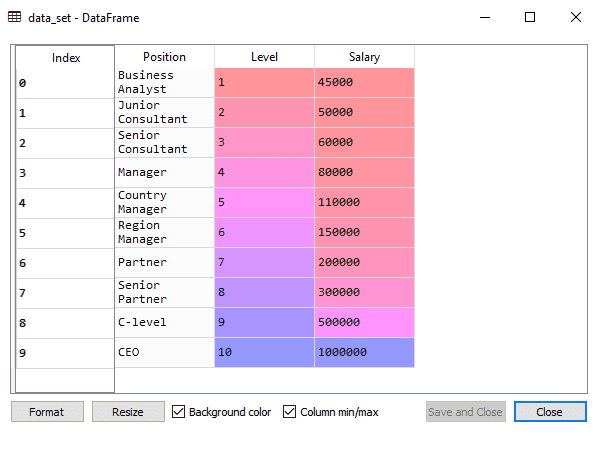There are three columns in the above output, as we can see (Positions, Levels, and Salaries). However, we'll simply look at two columns because Positions are comparable to levels or can be thought of as the encoded version of Positions.
Because the candidate has 4+ years of experience as a regional manager, he must be somewhere between levels 7 and 6, thus we'll anticipate the output for level 6.5.

## Building the Linear regression model:

We'll now create and fit a Linear regression model to the data. We'll use the Linear regression model as a starting point for creating polynomial regression and compare the outcomes. The code is as follows:

``````
#Fitting the Linear Regression to the dataset
from sklearn.linear_model import LinearRegression
lin_regs = LinearRegression()
lin_regs.fit(x,y)
```
```

Using the lin regs object of the LinearRegression class, we generated a Simple Linear model and fitted it to the dataset variables in the code above (x and y).

Output:
``````
LinearRegression(copy_X = True, fit_intercept=True, n_jobs = None, normalize=False)
```
```

## Building the Polynomial regression model:

We'll now construct the Polynomial Regression model, which will differ slightly from the Simple Linear model. Because the PolynomialFeatures class of the preprocessing library will be used here. This class is used to add some more features to our dataset.

``````
#Fitting the Polynomial regression to the dataset
from sklearn.preprocessing import PolynomialFeatures
poly_regs = PolynomialFeatures(degree = 2)
x_poly = poly_regs.fit_transform(x)
lin_reg_2 = LinearRegression()
lin_reg_2.fit(x_poly, y)
```
```

We used poly regs.fit transform(x) in the above lines of code because we are first turning our feature matrix into a polynomial feature matrix and then fitting it to the Polynomial regression model. The value of the parameter (degree= 2) is determined by our choosing. We can select it based on our Polynomial characteristics.
We will get another matrix x poly after running the code, which can be inspected under the variable explorer option: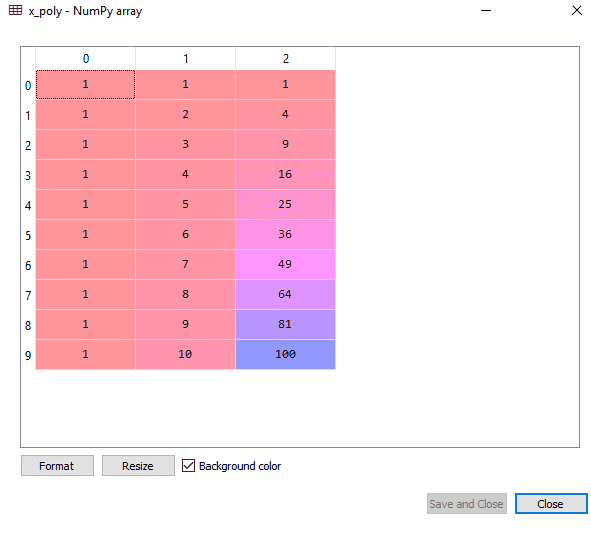Then, we have used another LinearRegression object, which is lin_reg_2, to fit our x_poly vector to the linear model.

Output:

``````
Out: LinearRegression(copy_X = True, fit_intercept = True, n_jobs = None, normalize = False)
```
```

## Visualizing the result for Linear regression:

Now, much as we did with Simple Linear Regression, we'll visualize the outcome for the Linear regression model. The code for it is as follows:

``````
#Visulaizing the result for Linear Regression model
mtp.scatter(x,y,color="blue")
mtp.plot(x,lin_regs.predict(x), color="red")
mtp.title("Bluff detection model(Linear Regression)")
mtp.xlabel("Position Levels")
mtp.ylabel("Salary")
mtp.show()
```
```

Output: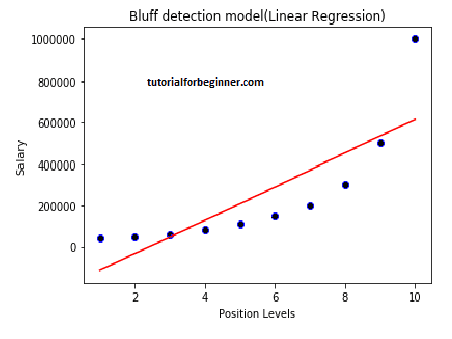The regression line is clearly separated from the datasets in the above output image. Predictions are represented by a red straight line, whereas actual values are represented by blue points. If we use this output to estimate the worth of a CEO, we get a pay of around 600000 dollars, which is significantly below the real value.

As a result, rather of a straight line, we require a curved model to suit the dataset.

## Visualizing the Polynomial Regression result

The output of the Polynomial regression model, whose code is slightly different from the above model, will be visualized below.
Code for this is given below:

``````
#Visulaizing the result for Polynomial Regression
mtp.scatter(x,y,color="blue")
mtp.plot(x, lin_reg_2.predict(poly_regs.fit_transform(x)), color="red")
mtp.title("Bluff detection model(Polynomial Regression)")

mtp.xlabel("Position Levels")
mtp.ylabel("Salary")
mtp.show()
```
```

In the code above, we have taken `lin_reg_2.predict(poly_regs.fit_transform(x)`, instead of x_poly, because we want a Linear regressor object to predict the polynomial features matrix.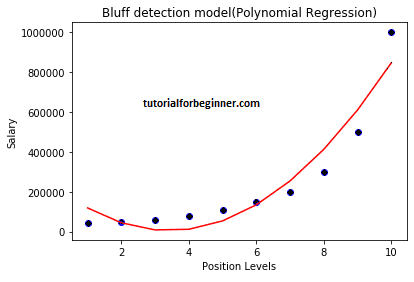The forecasts are close to the real numbers, as shown in the above output image. As we modify the degree, the plot above will change.

For a degree = 3
We can get a more accurate plot if we change the degree=3, as illustrated in the image below.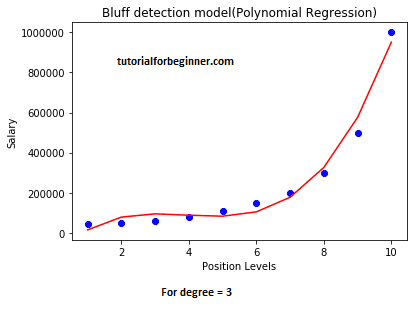As can be seen in the above output image, the forecasted salary for level 6.5 is around 170K\$-190K\$, implying that the prospective employee is telling the truth about his income.
Change the degree= 4 once more, and you'll have the most exact plot now. As a result, raising the degree of Polynomial will yield more accurate findings.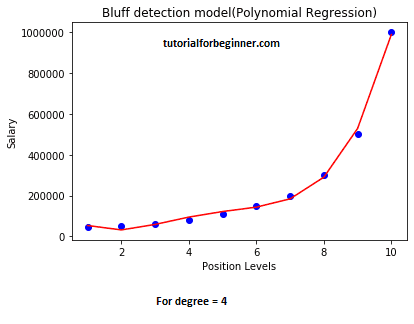## Using the Linear Regression model to predict the final result:

To determine if an employee is telling the truth or making a bluff, we will use the Linear regression model to predict the final outcome. So, we'll utilize the predict() method and pass the value 6.5 as a parameter. The code for it is as follows:

``````
lin_pred = lin_regs.predict([[6.5]])
print(lin_pred)
```
```

Output:
[330378.78787879]

Predicting the final result with the Polynomial Regression model:
Now, we'll use the Polynomial Regression model to forecast the final output and compare it to the Linear model. The code for it is as follows:

``````
poly_pred = lin_reg_2.predict(poly_regs.fit_transform([[6.5]]))
print(poly_pred)
```
```

Output:
[158862.45265153]

As we can see, the Polynomial Regression's projected output is [158862.45265153], which is significantly closer to the real value, so we can conclude that the future employee is correct.